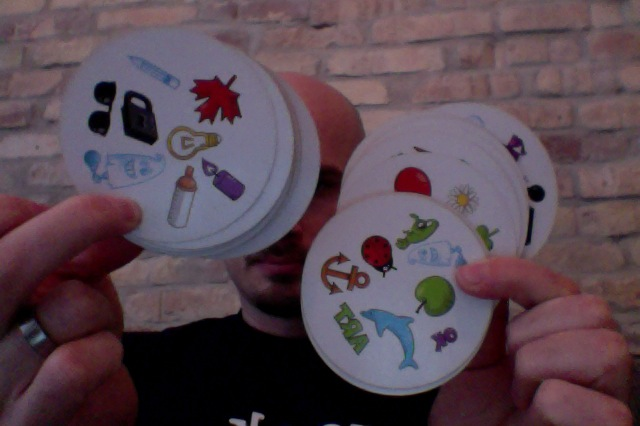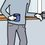# Spot It problem, version 2The game "Spot It!" consists of a deck of cards.

In the original version of the game, every card has 8 symbols on it. If you pick any two cards, exactly one symbol will be the same on the cards.

In the kids version of the game, every card has 6 symbols instead, but you still have exactly one match of symbols if you pick any two cards.

You may also assume that the deck contains the same number of every symbol (that is, no symbol is more common than another).

Suppose you want to create a more complex version of the game, where you have N symbols on each card and every two cards share exactly M symbols.

a) Is this possible?

In a deck with N symbols on each card, and each pair of cards share M symbols:

b) How many cards will there be at most?

c) How many symbols will there be in total?

Note: The combination of symbols matching two cards should, if possible, be different for every two cards. (Thanks for Utkarsh Dwivedi for the question leading to this clarification.)Note by Johan Falk
6 years, 10 months ago

This discussion board is a place to discuss our Daily Challenges and the math and science related to those challenges. Explanations are more than just a solution — they should explain the steps and thinking strategies that you used to obtain the solution. Comments should further the discussion of math and science.

When posting on Brilliant:

• Use the emojis to react to an explanation, whether you're congratulating a job well done , or just really confused .
• Ask specific questions about the challenge or the steps in somebody's explanation. Well-posed questions can add a lot to the discussion, but posting "I don't understand!" doesn't help anyone.
• Try to contribute something new to the discussion, whether it is an extension, generalization or other idea related to the challenge.

MarkdownAppears as
*italics* or _italics_ italics
**bold** or __bold__ bold
- bulleted- list
• bulleted
• list
1. numbered2. list
1. numbered
2. list
Note: you must add a full line of space before and after lists for them to show up correctly
paragraph 1paragraph 2

paragraph 1

paragraph 2

[example link](https://brilliant.org)example link
> This is a quote
This is a quote
    # I indented these lines
# 4 spaces, and now they show
# up as a code block.

print "hello world"
# I indented these lines
# 4 spaces, and now they show
# up as a code block.

print "hello world"
MathAppears as
Remember to wrap math in $$ ... $$ or $ ... $ to ensure proper formatting.
2 \times 3 $2 \times 3$
2^{34} $2^{34}$
a_{i-1} $a_{i-1}$
\frac{2}{3} $\frac{2}{3}$
\sqrt{2} $\sqrt{2}$
\sum_{i=1}^3 $\sum_{i=1}^3$
\sin \theta $\sin \theta$
\boxed{123} $\boxed{123}$

Sort by:

Well!!!

The problem is more complex than I thought....

Let us try to find all the solutions here..

First let me try to prove that for any value of N, there can be at least two values of M , namely 1 and {N-1}

Let there be D cards in the deck, each with N Symbols. Let any pair of cards have M symbols in common. Let the total symbols be S.

If there is any case of S = N, then two cards are enough!! To avoid the aforementioned contradiction, let us say that there is atleast one more symbol other than N [i.e] D > 2

Now, take the first card with N Symbols. We can have C [ N , M] more cards with suitable combination.

==> Max. No. of Cards D = C [ N , M] + 1 .................{1}

Now, Least Value of D is given by least value of C [ N,M ]

C [N,M] = 1 ==> M = {0, N} ==> D =2 ... Contradicts with our assumption..

C [N,M] = N ==> M = { 1, N-1 } ==> D = N+1 ...............................{2}

Hence, we have proved that for any value of N, atleast two values of M , i.e M = { 1, N-1 } are available

Again, we know that each card has [N-M] distinct symbols from first one...

Hence, No. of Symbols S = C [ D , N - M ] ...............................{3}

We can get S in another method also. The first card have N distinct Symbols. The second card has [N-M] distinct symbols. The third card has [N-2M] distinct symbols and so on....

Let Q {N,M} and R {N,M} be the Quotient and remainder respectively, when N is divided by M.

We know that A = B . Q {A,B} + R {A,B}

Now, Total No. of symbols S = N + [N-M] + [N-2M] + .... + [N - Q {N,M} M]

= N [1+1+..... {Q {N,M} + 1} times ] - M [1+2+...+ Q {N,M} ]

= N [ Q {N,M} +1 ] - M [ Q {N,M} ] [ Q {N,M} + 1 ] / 2 = [ Q {N,M} + 1 ] [ 2 N - M . Q {N,M} ] / 2

==> S = [ Q {N,M} + 1 ] [ N + R {N,M} ] / 2 .......................{4}

To find S for the cases M = { 1, N-1 }

M = 1 ==> S = N [ N+1 ] / 2
M = N-1 ==> S = N+1

Both methods give the same result. Hence, the result is the optimal most one.

To Conclude,

a) For any N symbols per card , there exists atleast two natural numbers M such that any pair of cards have exactly M symbols in common

M = { 1, N-1 }

b) No. of cards = N+1 , for both the cases

c) No. of Symbols

Atmost S = N [ N+1 ] / 2 for M = 1

The other result M = { N-1 } gives us [ N+1 ] only

Further findings:

Here are few more findings which I found during attempt to solution. But, I donot know how to prove them:

1. For any prime No. P, only two solutions are possible M = { 1, P-1 }

2. For any N, the only co prime for which a solution is available is M = N-1 {i.e} Any set of Co primes N , M do not have a solution unless M = N-1

3. Non Co-prime sets N, M

If N = f A and M = f B, f being the H.C.F of N and M , then the problem should be rephrased as f { A , B } . Now, the problem is to be solved as above.

No. of Cards for { N , M } = No. of Cards for { A , B }

No. of Symbols for { N , M } = f * [ No. of Symbols for { A , B } ]

P.S: Thank you for the problem which occupied my day. I hope that I have answered correctly.

- 6 years, 10 months ago

Can the same group of symbols be common to more than two cards ?

- 6 years, 10 months ago

Good question. I would say no, and I should update the question to say that not only every symbol should be equally frequent – but also every combination of symbols between cards. (I'm not sure this is possible, though…)

Thanks!

- 6 years, 10 months ago